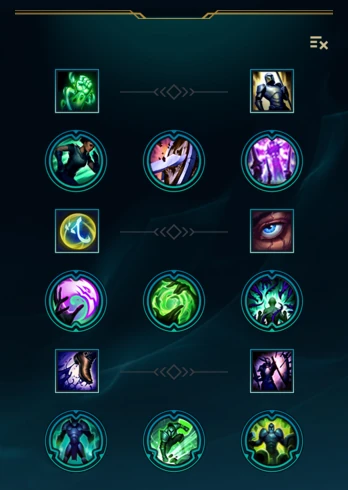League of Legends Wiki

Come join the LoL Wiki community Discord server!"You belong in a museum!" This article or section may contain obsolete information, but exists here for historical purposes.

Resolve TreeOther Mastery Trees:FerocityCunning
Fearless is a tier 4 Resolve mastery with 1 rank. 

Recursion

• Denote the base armor as a, bonus armor (without counting the above multipliers) as b, bonus armor percentage increase due to as x, total armor percentage increase due to as y, and flat bonus from as c
• y = 10% = 0.1
• The resultant armor can be calculated as follows:
• Define two sequences S = {sn} and T = {tn}, where n = 0, 1, 2, ...
• s0 = a + b + c, t0 = 0
• s1 = (a + b)y, t1 = (b + c)x
• For n ≥ 2, sn = tn − 1y, tn = sn − 1x
• The resultant armor is the sum of all terms in S and T.
• This gives the formula Resultant armor = [a(1 + y) + b(1 + x)(1 + y) + c(1 + x)] ÷ (1 − xy)
• Same works for magic resistance.

Patch History

V7.22
• Removed due to Season 2018.
V7.4
• Flat bonus resistances reduced to 1.5 − 27 (based on level) from 2 − 36 (based on level)
V6.22